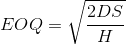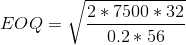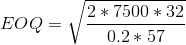##### Question

In: Operations Management

# Ergonomics Inc. sells ergonomically designed office chairs. The company has the following information: Average demand =...

Ergonomics Inc. sells ergonomically designed office chairs. The company has the following information: Average demand = 30 units per day Average lead time = 37 days Item unit cost = $57 for orders of less than 270 units Item unit cost =$56 for orders of 270 units or more Ordering cost = $32 Inventory carrying cost = 20% The business year is 250 days Assume there is no uncertainty at all about the demand or the lead time. a. Calculate EOQ if unit cost is$57 and $56. (Note: These EOQs do not need to be feasible in their price range.) b. Calculate annual ordering costs for each alternative? c. Calculate annual inventory carrying costs for each alternative? d. Calculate annual product costs for each alternative? e. What will be the total costs for each alternative? (Round your answers to 2 decimal places.) f. Based on your analysis, how many chairs should they order at a time? g. How much the firm can save annually by using the order quantity in Part f. instead of the first EOQ shown in Part a? ## Solutions ##### Expert Solution Average demand per day = 30 units The business year = 250 days Annual Demand, D = 250 *30 = 7500 units Average lead time = 37 days Unit cost =$57 for orders of less than 270 units

unit cost = $56 for orders of 270 units or more Ordering cost, S =$32

Inventory carrying cost = 20%

For orders of less than 270 units, Holding Cost per unit, H = 20% *57

For orders of 270 units or more, Holding Cost per unit, H = 20% *56@56 unit price, EOQ =  207.0197@57 unit priceEOQ = 205.1956

 @56 @57 EOQ 270.000 205.196 No.of Orders = 7500/EOQ 27.77778 36.55048 Order Cost = No.of orders*32 888.8889 1169.615 Purchase cost = 7500*unit price 420000 427500 Annual Holding Cost = (EOQ/2)*H 1512 1169.615 422400.9 429839.2

b)

Annual Ordering Cost @ 56 = $888.88 Annual Ordering Cost @ 57 =$ 1169.615

c)

Annual inventory carrying cost @ 56 = $1512 Annual inventory carrying cost @ 57 =$ 1169.615

d)

Annual product cost @ 56 = 420000

Annual product cost @ 57 = 427500

e)

Total costs @56 = 422400.9

Total costs @57 = 429839.2

f)

Ordering the chair at the discount price has less total cost. Hence 270 units should be ordered.

g)

 @56 @57 @56 EOQ 270.000 205.196 207.020 Initial EOQ Quantity No.of Orders = 7500/EOQ 27.77778 36.55048 36.22844 Order Cost = No.of orders*32 888.8889 1169.615 1159.31 Purchase cost = 7500*unit price 420000 427500 420000 Annual Holding Cost = (EOQ/2)*H 1512 1169.615 1159.31 422400.9 429839.2 422318.6

Saved at discount by ordering 270 units = 429839.2-422400.9 = 7438.342

The EOQ for 56 unit price is 207.02.

By ordering 270 units to avail discount, 429839.2-422318.6 = 82.26 is lost when compared with part a.

## Related Solutions

##### Office Furniture Systems, Inc. sells chairs for $150. The unit variable cost per unit is$65....
Office Furniture Systems, Inc. sells chairs for $150. The unit variable cost per unit is$65. Fixed costs total $18,700. Required: a. What is the contribution margin per unit? b. What is the breakeven point in units? c. How many units must be sold to earn a pretax income of$45,000? d.   What is the margin of safety, assuming 700 units are sold?